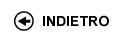Politecnico di Torino
Anno Accademico 2014/15
01PDDOV, 01PDDQW
Identification and control methodologies
Corso di Laurea Magistrale in Ingegneria Informatica (Computer Engineering) - Torino
Corso di Laurea Magistrale in Ingegneria Meccatronica (Mechatronic Engineering) - Torino
 Docente Qualifica Settore Lez Es Lab Tut Anni incarico Taragna MicheleAC ING-INF/04 36 8 16 0 4
 SSD CFU Attivita' formative Ambiti disciplinari ING-INF/04 6 C - Affini o integrative A11
Esclusioni:
01NRE
 Presentazione The course is taught in English. The purpose of this course is to provide basic methodologies and software tools for building mathematical models of static or dynamic systems from experimental data, suitable for designing model-based control systems in presence of constraints. Risultati di apprendimento attesi The student shall acquire the following knowledge and develop the following abilities: - Knowledge of main methods and software tools for building mathematical models (based on physical-laws or in form of difference equations, input-state-output equations or transfer functions) of linear and nonlinear (static or dynamic) systems - Knowledge of main methods and software tools for evaluating estimate reliability and model quality - Knowledge of basic theoretical properties of main methods for building mathematical models of static or dynamic systems - Skill in building mathematical models of linear and nonlinear systems exploiting both physical information and experimental data - Skill in evaluating estimate reliability and model quality - Knowledge of design techniques of model-based control systems in presence of constraints Prerequisiti / Conoscenze pregresse The following notions are essential: knowledge of the representations of linear dynamic systems (input-state-output equations, transfer functions) and of their fundamental properties (stability, controllability, observability); essentials of probability theory and statistics; basic concepts of linear algebra and Zeta transform. The knowledge of the MATLAB software environment is required. Programma Course topics and relative devoted time: - Introduction to estimation and prediction problems. Main statistical estimation methods (least squares, weighted least-squares, maximum likelihood estimators, Bayesian estimators) and their basic properties (correctness, consistency, efficiency), with evaluation of parametric estimation error (18 hours) - Set-membership estimation theory for different norm assumptions on noise, with evaluation of Estimate Uncertainty Sets and Intervals. Optimal and Central estimates, with evaluation of Feasible Parameter Sets and Parameter Uncertainty Intervals (7 hours) - Introduction to Kalman filtering problem: dynamic one-step and multi-step predictors, dynamic optimal filter, steady-state one-step predictor and filter, nonlinear predictors and filters (10 hours) - Identification of linear dynamic systems from input-output measurements: FIR, ARX, ARMAX and OE models. Predictive approach and models in predictor form. Asymptotic analysis of prediction-error identification methods. Least-squares method: probabilistic analysis, persistence of excitation, practical procedure. Recursive least-squares methods. Model structure selection and validation (whiteness test and residual analysis; FPE, AIC and MDL criteria) (17 hours) - Identification of nonlinear dynamic systems from input-output measurements: statistical and set-membership methods. Neural networks: approximation properties, learning (5 hours) - Introduction to optimal control problem in presence of constraints on input, state and output variables. Introduction to Model Predictive Control (MPC) and definition of the "receding horizon" strategy (3 hours) Organizzazione dell'insegnamento Exercise sessions are focused on the development of both academic and applicative examples. Some other sessions (18 hours) are carried out in computer laboratories and are focused on modelling real-world static or dynamic systems (position transducer, hair dryer, water heater) and on Kalman predictor and filter design and simulation for a given linear dynamic system, using MATLAB toolboxes (Control System, System Identification, Neural Network based System Identification). Testi richiesti o raccomandati: letture, dispense, altro materiale didattico The following textbooks have been mainly addressed in the organization of the course: - S. Bittanti, "Teoria della Predizione e del Filtraggio", VII edition, Pitagora Editrice Bologna, 2004 (in Italian) - S. Bittanti, "Identificazione dei Modelli e Sistemi Adattativi", VI edition, Pitagora Editrice Bologna, 2004 (in Italian) - T. Kailath, A. H. Sayed, B. Hassibi, "Linear Estimation", Prentice Hall, Upper Saddle River, N.J. (U.S.A.), 2000 - L. Ljung, "System Identification: Theory for the User", II edition, Prentice Hall PTR, Upper Saddle River, N.J. (U.S.A.), 1999 - L. Ljung, "System Identification Toolbox User’s Guide", The MathWorks Inc., Natick, MA (U.S.A.), 1988-1997 On the course web page, teaching material is available about specific issues addressed in the course, like lecture slides and laboratory exercises with proposed solutions. Criteri, regole e procedure per l'esame The final assessment consists of a written test, about three hours long, performed in the computer laboratory in order to use MATLAB tools. The examination is typically made of a model building practice of an unknown system starting from given data and a second exercise on Kalman predictor and/or filter design and simulation for a given linear dynamic system. Orario delle lezioni Statistiche superamento esami Programma definitivo per l'A.A.2014/15© Politecnico di Torino
Corso Duca degli Abruzzi, 24 - 10129 Torino, ITALY